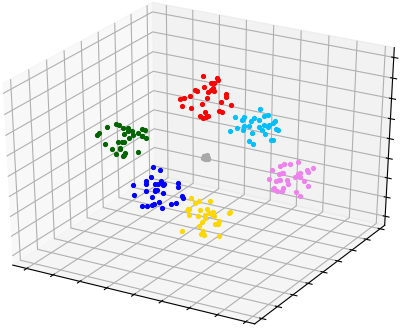pyclustering.cluster.silhouette.silhouette_ksearch Class Reference

Represent algorithm for searching optimal number of clusters using specified K-algorithm (K-Means, K-Medians, K-Medoids) that is based on Silhouette method. More...

## Public Member Functions

def __init__ (self, data, kmin, kmax, kwargs)
Initialize Silhouette search algorithm to find out optimal amount of clusters. More...

def process (self)
Performs analysis to find optimal amount of clusters. More...

def get_amount (self)
Returns optimal amount of clusters that has been found during analysis. More...

def get_score (self)
Returns silhouette score that belongs to optimal amount of clusters (k). More...

def get_scores (self)
Returns silhouette score for each K value (amount of clusters). More...

## Detailed Description

Represent algorithm for searching optimal number of clusters using specified K-algorithm (K-Means, K-Medians, K-Medoids) that is based on Silhouette method.

This algorithm uses average value of scores for estimation and applicable for clusters that are well separated. Here is an example where clusters are well separated (sample 'Hepta'):

from pyclustering.cluster import cluster_visualizer
from pyclustering.cluster.center_initializer import kmeans_plusplus_initializer
from pyclustering.cluster.kmeans import kmeans
from pyclustering.cluster.silhouette import silhouette_ksearch_type, silhouette_ksearch
from pyclustering.samples.definitions import FCPS_SAMPLES
search_instance = silhouette_ksearch(sample, 2, 10, algorithm=silhouette_ksearch_type.KMEANS).process()
amount = search_instance.get_amount()
scores = search_instance.get_scores()
print("Scores: '%s'" % str(scores))
initial_centers = kmeans_plusplus_initializer(sample, amount).initialize()
kmeans_instance = kmeans(sample, initial_centers).process()
clusters = kmeans_instance.get_clusters()
visualizer = cluster_visualizer()
visualizer.append_clusters(clusters, sample)
visualizer.show()

Obtained Silhouette scores for each K:

Scores: '{2: 0.418434, 3: 0.450906, 4: 0.534709, 5: 0.689970, 6: 0.588460, 7: 0.882674, 8: 0.804725, 9: 0.780189}'

K = 7 has the bigger average Silhouette score and it means that it is optimal amount of clusters:Silhouette ksearch's analysis with further K-Means clustering (sample 'Hepta').
silhouette_ksearch_type

Definition at line 305 of file silhouette.py.

## ◆ __init__()

 def pyclustering.cluster.silhouette.silhouette_ksearch.__init__ ( self, data, kmin, kmax, kwargs )

Initialize Silhouette search algorithm to find out optimal amount of clusters.

Parameters
 [in] data (array_like): Input data that is used for searching optimal amount of clusters. [in] kmin (uint): Amount of clusters from which search is performed. Should be equal or greater than 2. [in] kmax (uint): Amount of clusters to which search is performed. Should be equal or less than amount of points in input data. [in] **kwargs Arbitrary keyword arguments (available arguments: 'algorithm').

Keyword Args:

• algorithm (silhouette_ksearch_type): Defines algorithm that is used for searching optimal number of clusters (by default K-Means).
• ccore (bool): If True then CCORE (C++ implementation of pyclustering library) is used (by default True).

Definition at line 350 of file silhouette.py.

## ◆ get_amount()

 def pyclustering.cluster.silhouette.silhouette_ksearch.get_amount ( self )

Returns optimal amount of clusters that has been found during analysis.

Returns
(uint) Optimal amount of clusters.
process

Definition at line 435 of file silhouette.py.

## ◆ get_score()

 def pyclustering.cluster.silhouette.silhouette_ksearch.get_score ( self )

Returns silhouette score that belongs to optimal amount of clusters (k).

Returns
(float) Score that belong to optimal amount of clusters.
process, get_scores

Definition at line 447 of file silhouette.py.

## ◆ get_scores()

 def pyclustering.cluster.silhouette.silhouette_ksearch.get_scores ( self )

Returns silhouette score for each K value (amount of clusters).

Returns
(dict) Silhouette score for each K value, where key is a K value and value is a silhouette score.
process, get_score

Definition at line 459 of file silhouette.py.

## ◆ process()

 def pyclustering.cluster.silhouette.silhouette_ksearch.process ( self )

Performs analysis to find optimal amount of clusters.